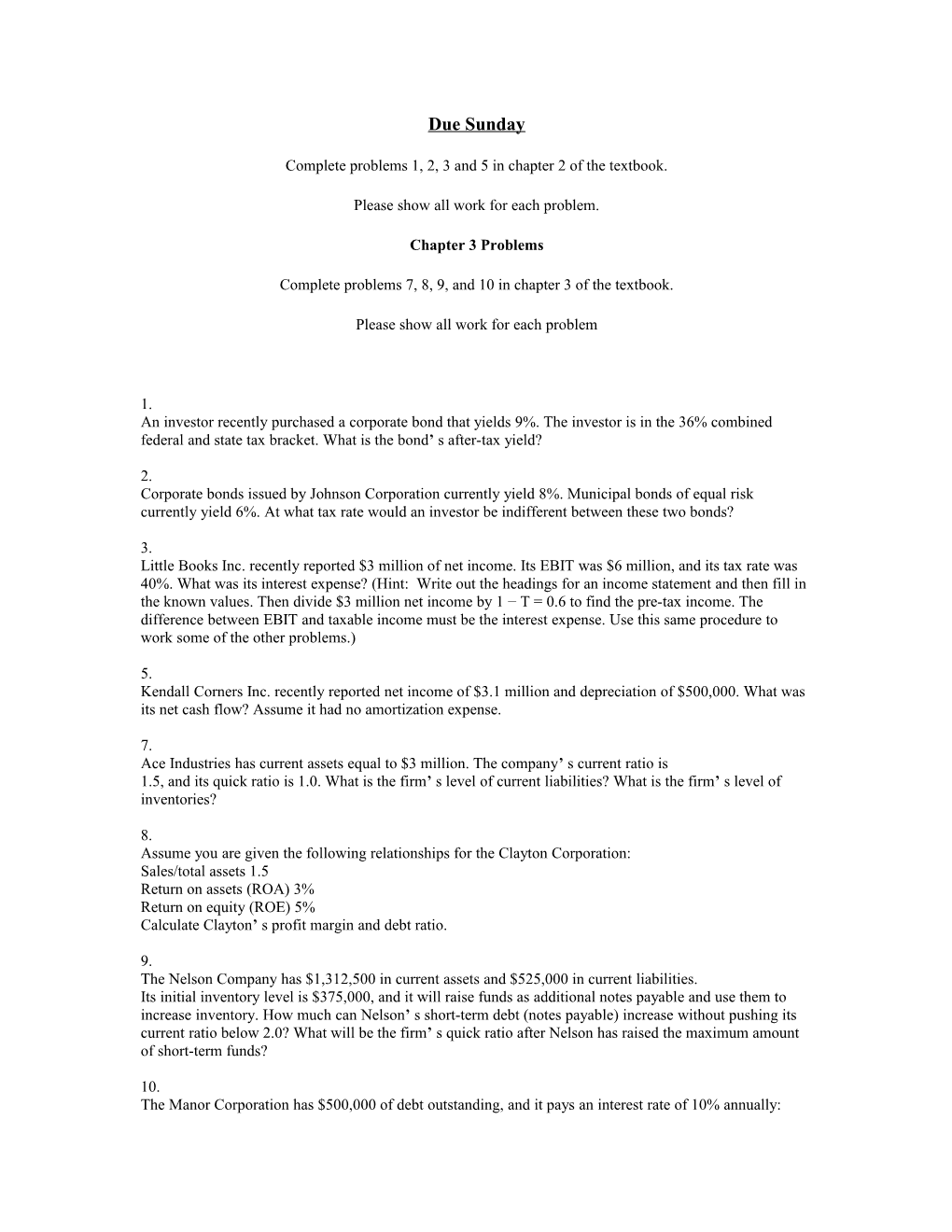# Complete Problems 1, 2, 3 and 5 in Chapter 2 of the TextbookDue Sunday

Complete problems 1, 2, 3 and 5 in chapter 2 of the textbook.

Please show all work for each problem.

Chapter 3 Problems

Complete problems 7, 8, 9, and 10 in chapter 3 of the textbook.

Please show all work for each problem

1.

An investor recently purchased a corporate bond that yields 9%. The investor is in the 36% combined federal and state tax bracket. What is the bond’ s after-tax yield?

2.

Corporate bonds issued by Johnson Corporation currently yield 8%. Municipal bonds of equal risk currently yield 6%. At what tax rate would an investor be indifferent between these two bonds?

3.

Little Books Inc. recently reported \$3 million of net income. Its EBIT was \$6 million, and its tax rate was 40%. What was its interest expense? (Hint: Write out the headings for an income statement and then fill in the known values. Then divide \$3 million net income by 1 − T = 0.6 to find the pre-tax income. The difference between EBIT and taxable income must be the interest expense. Use this same procedure to work some of the other problems.)

5.

Kendall Corners Inc. recently reported net income of \$3.1 million and depreciation of \$500,000. What was its net cash flow? Assume it had no amortization expense.

7.

Ace Industries has current assets equal to \$3 million. The company’ s current ratio is

1.5, and its quick ratio is 1.0. What is the firm’ s level of current liabilities? What is the firm’ s level of inventories?

8.

Assume you are given the following relationships for the Clayton Corporation:

Sales/total assets 1.5

Return on assets (ROA) 3%

Return on equity (ROE) 5%

Calculate Clayton’ s profit margin and debt ratio.

9.

The Nelson Company has \$1,312,500 in current assets and \$525,000 in current liabilities.

Its initial inventory level is \$375,000, and it will raise funds as additional notes payable and use them to increase inventory. How much can Nelson’ s short-term debt (notes payable) increase without pushing its current ratio below 2.0? What will be the firm’ s quick ratio after Nelson has raised the maximum amount of short-term funds?

10.

The Manor Corporation has \$500,000 of debt outstanding, and it pays an interest rate of 10% annually: Manor’ s annual sales are \$2 million, its average tax rate is

30%, and its net profit margin on sales is 5%. If the company does not maintain a

TIE ratio of at least 5 to 1, then its bank will refuse to renew the loan and bankruptcy will result. What is Manor’ s TIE ratio?